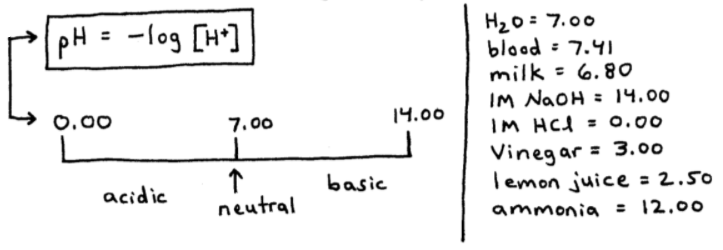# S14E3 - The pH Scale and pH Calculations

## Water is an Amphoteric Substance

Amphoteric  =  it can act as either an acid or a base.

Besides H2O, ammonia (NH3) is also an amphoteric substance.  Let's take a look at the autoionization of water...

### Autoionization of water

Water is capable of ionizing itself, as shown below:

ex:    H2O  +  H2O  ⇌  H3O+  +  OH-From above, we have:

2H2O (l)   ⇌   H3O+ (aq)   +   OH- (aq)

so,  the Dissociation Constant for Water is:
Kw  =  [H3O+] [OH-]

or,  Kw  =  [H+] [OH-]  =  1.0 x 10-14  (at 25°C)

-----

In pure water, or a neutral solution at 25°C,

[H+]  =  [OH-]  =  1.0 x 10-7 M

➞  in an acidic solution,  [H+]  >  [OH-]
but  Kw  =  1.0 x 10-14  still.

➞  in a basic solution,  [OH-]  >  [H+]
but  Kw  =  1.0 x 10-14  still.

-----

ex:  Calculate the [H+] and [OH-] concentrations.

a.  1.0 x 10-5 M OH-

➞  Kw  =  [H+] [OH-]  =  1.0 x 10-14

➞  Kw  =  [H+] (1.0 x 10-5)  =  1.0 x 10-14

➞  [H+]  =  1.0 x 10-8 M  ➞  [OH-]  >  [H+]
so the solution is basic.

b.  10.0 M OH-

➞  Kw  =  [H+] [OH-]  =  1.0 x 10-14

➞  Kw  =  (10.0) [OH-]  =  1.0 x 10-14

➞  [OH-]  =  1.0 x 10-15 M  ➞  [H+]  >>  [OH-]
so the solution is very acidic.

=====

## The pH Scale

The pH scale is a convenient way to represent a solution's acidity.  The pH equation is shown below, and the scale goes from 0.00 to 14.00.

### The pH Equation and pH ScaleAs you can see in the image above, pH values less than 7.00 are acidic, while pH values above 7.00 are basic.  A pH value equal to 7.00 would be neutral.

ex:  Calculate the pH of the following solutions.

a.  1.0 x 10-3 M  H+

➞  pH  =  - log [H+]  =  - log (1.0 x 10-3)

➞  pH  =  3.00

b.  1.0 x 10-5 M  H+

➞  pH  =  - log [H+]  =  - log (1.0 x 10-5)

➞  pH  =  5.00

-----
shortcut:  when you're asked to find the pH of a solution having a concentration of 1.0 x 10-z, the pH = "z"

ex:  1 x 10-9 M  H+  ➞  pH = 9.00
ex:  1.0 x 10-8 M  H+  ➞  pH = 8.00
ex:  2.3 x 10-7 M  H+  ➞  no shortcut

=====

### The pOH Scale

If you know the equation for pH, then you can probably guess the pOH equation.  Below you can see how pH, pOH, and pKw are all related...ex Calculate the pH and pOH of a 2.6 x 10-12 M OH- solution.
_________

"2.6" is the base number, so we can't do the shortcut calculation.  Since we're given the [OH-], we should calculate the pOH first:

➞  pOH  =  - log [OH-]
pOH  =  - log (2.6 x 10-12)
pOH  =  11.58

Now, use the pOH value of 11.58 to solve for pH...

➞  pH  +  pOH  =  14.00
pH  +  11.58  =  14.00
pH  =  2.42

-----

ex:  The pH of blood is 7.41.  Find the pOH, [H+], and [OH-]
_________